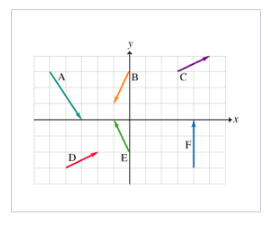# Problem: Adding and Subtracting Vectors Conceptual QuestionSix vectors ( A to F) have the magnitudes and directions indicated in the figure. Which two vectors, when added, will have the largest (positive) x component?1. C &amp; E2. E &amp; F3. A &amp; F4. C &amp; D5. B &amp; D

###### FREE Expert Solution

A = 2i - 3j

B = -1i - 2j

C = 2i + 1j

D = 2i + 1j

E = -1i + 2j

F = 0 i + 3j

Sum of x-componets:

1. 2i - 1i = 1i

2. -1i + 0i = -1i

81% (352 ratings)###### Problem Details

Adding and Subtracting Vectors Conceptual Question

Six vectors ( A to F) have the magnitudes and directions indicated in the figure.Which two vectors, when added, will have the largest (positive) x component?

1. C & E

2. E & F

3. A & F

4. C & D

5. B & D

Frequently Asked Questions

What scientific concept do you need to know in order to solve this problem?

Our tutors have indicated that to solve this problem you will need to apply the Adding Vectors by Components concept. You can view video lessons to learn Adding Vectors by Components. Or if you need more Adding Vectors by Components practice, you can also practice Adding Vectors by Components practice problems.